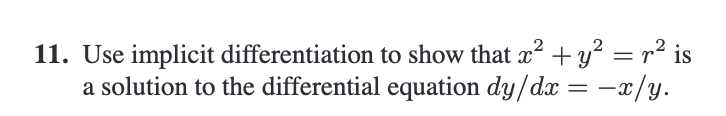Home / Expert Answers / Calculus / 11-use-implicit-differentiation-to-show-that-x2-y2-r2-is-a-solution-to-the-differential-equation-pa255

# (Solved): 11. Use implicit differentiation to show that x2+y2=r2 is a solution to the differential equation ...11. Use implicit differentiation to show that is a solution to the differential equation .

We have an Answer from Expert